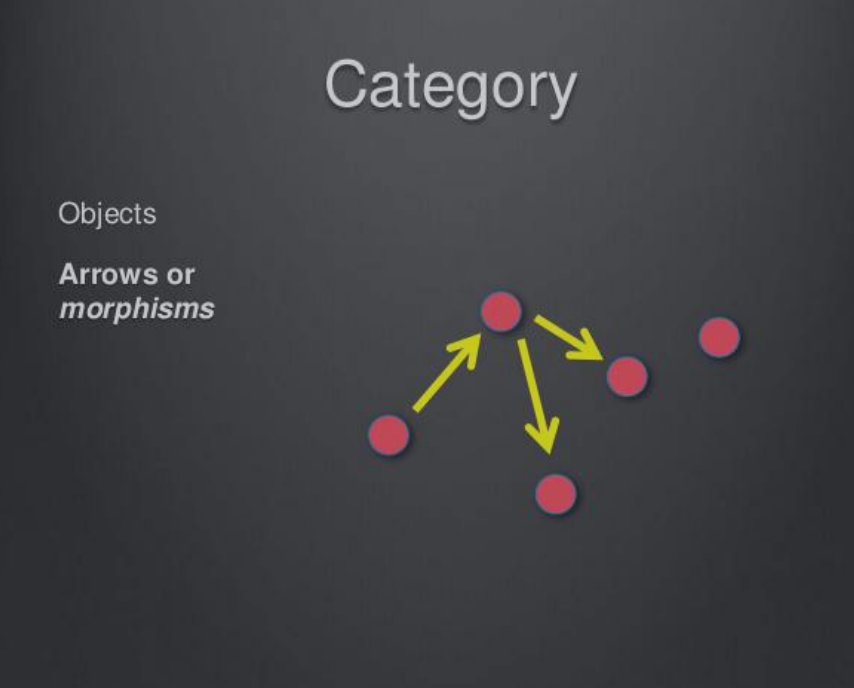# 函数式编程

## 范畴论 Category Theory​• 所有成员是一个集合
• 变形关系是函数

## 函数式编程基础概念​

JavaScript 是披着 c 外衣的 LISP

### 函数是一等公民​

map 和 reduce 它们是最常用的函数式编程的方法

#### 特点​

• 函数是“一等公民”
• 只用表达式,不使用语句; 没有 if,else 什么的东西,用数学的思维解决它
• 没有副作用
• 不修改状态
• 引用透明(函数运行只依靠参数,且相同的输入总是获得相同的输出)

## 函数式编程核心概念​

### 纯函数​

``let arr = [1,2,3]console.log(arr.slice(0,3)) //[1,2,3]console.log(arr.slice(0,3)) //[1,2,3]console.log(arr.slice(0,3)) //[1,2,3]//slice 就是纯函数;console.log(arr.splice(0,3)) //[1,2,3]console.log(arr.splice(0,3))//[]console.log(arr.splice(0,3))//[]//splice 就改变了 arr,这个 arr 就被污染了;相同的输入,输出结果不同``

#### 优缺点​

``// area 1import _ from 'lodash';var sin = _.memorize(x => Math.sin(x));// 第一次缓存会慢一些var a = sin(1);// 第二次有了缓存，速度极快var b = sin(1);// area 2var min = 18;// 非纯函数var checkage = age => age > min;// 纯函数var checkage = age => age > 18``

### 纯度和幂等性​

#### 幂等性​

``Math.abs(Math.abs(-42))``

### 偏应用函数​

``// 带一个函数参数和该函数的部分参数const parital = (f, ...args) => {  (...moreArgs) => {    f(...args, ...moreArgs)  }}const add3(a, b, c) => a + b + c// 偏应用 2 和 3 到 add3 给你的一个单参数的函数const fivePlus = partial(add3, 2, 3)fivePlus(4)// bind 实现const add1More = add3.bind(null, 2, 3) // (c)=> 2 + 3 + c``

### 函数的柯里化​

``var checkage = min => (age => age > min)var ck18 = checkage(18)ck18(20)``

#### 函数的反柯里化​

``// 柯里化// 柯里化之前function add (x, y) {  return x + y}add(1, 2) // 3// 柯里化之后function addX(x) {  return function(y) {    return x + y  }}add(1)(2) // 3// 反柯里化Function.prototype.unCurring = function() {  var self = this  return function () {    var obj = Array.prototype.shift.call(arguments)    return self.apply(obj, arguments)  }}var push = Array.prototype.push.unCurrying()var obj = {}push(obj, 'first', 'two')console.log(obj)``

``    const setTimeoutWraper = (timeout,fn)=>{        setTimeout(fn,timeout)    }    const delayTenMs = curring(setTimeoutWraper)(10)    delayTenMs(()=>console.log('1'));    delayTenMs(()=>console.log(2))``

setTimeoutWraper 显得多余,这时候我们就可以使用偏函数，使用 curry 和partial 是为了让函数参数或者函数设置变得更加简单和强大， curry 和 partial实现方式可以参考 lodash

### 函数组合​

#### 函数组合子​

compose 函数只能组合接受一个参数的函数，类似于 filter、map、接受两个参数（投影函数：总是在应用转换操作，通过传入高阶参数后返回数组），不能被直接组合可以借助偏函数包裹后继续组合

• 辅助组合子

nothing(没有)、identity（照旧）、defaultTo(默许)、always（恒定）

• 函数组合子

• 谓语组合子

• 其他

### Of 方法​

``Functor.of = function(val) {  return new Functor(val);};``

``Functor.of(2).map(function(two) {  return two + 2;});// Functor(4)``

### Maybe 函子​

Maybe 函子就是为了解决这一类问题而设计的。简单说，它的 map 方法里面设置了空值检查。

``class Maybe extends Functor {  constructor(value) {    super();    this.val = value;  }  isnothing() {    return !!!this.val;  }  map(f) {    if (this.isnothing()) {      // 如果没有值，不执行变形函数，直接返回一个新函子 null。      return Maybe.of(null);    } else {      return Maybe.of(f(this.val));    }  }}``

### Either 函子​

Either 函子内部有两个值：左值（Left）和右值（Right）。右值是正常情况下使用的值，左值是右值不存在时使用的默认值。

``class Either extends Functor {  constructor(value) {    super();    this.val = value;  }  isnothing() {    return !!!this.val;  }  map(left, right) {    if (this.isnothing()) {      return Either.of(left(null));    } else {      return Either.of(right(this.val));    }  }}``

### AP 函子​

``function addTwo(x) {  return x + 2;}const A = Functor.of(2);const B = Functor.of(addTwo);``

ap 是 applicative（应用）的缩写。凡是部署了 ap 方法的函子，就是 ap 函子。

``class Ap extends Functor {  constructor(value) {    super();    this.val = value;  }  ap(F) {    return Ap.of(this.val(F.val));  }}``

``Ap.of(addTwo).ap(Functor.of(2));// Ap(4)``

ap 函子的意义在于，对于那些多参数的函数，就可以从多个容器之中取值，实现函子的链式操作

``function add(x) {  return function(y) {    return x + y;  };}Ap.of(add)  .ap(Maybe.of(2))  .ap(Maybe.of(3));// Ap(5)``

``Ap.of(add(2)).ap(Maybe.of(3));``

``Maybe.of(Maybe.of(Maybe.of({ name: 'Mulburry', number: 8402 })));``

Monad 函子的作用是，总是返回一个单层的函子。它有一个 flatMap 方法，与 map 方法作用相同，唯一的区别是如果生成了一个嵌套函子，它会取出后者内部的值，保证返回的永远是一个单层的容器，不会出现嵌套的情况。

``class Monad extends Functor {  join() {    return this.val;  }  flatMap(f) {    return this.map(f).join();  }}``

### IO 函子​

I/O 是不纯的操作，普通的函数式编程没法做，这时就需要把 IO 操作写成 Monad 函子，通过它来完成。

``var fs = require('fs');var readFile = function(filename) {  return new IO(function() {    return fs.readFileSync(filename, 'utf-8');  });};var print = function(x) {  return new IO(function() {    console.log(x);    return x;  });};``

``readFile('./user.txt').flatMap(print);``

``var tail = function(x) {  return new IO(function() {    return x[x.length - 1];  });};readFile('./user.txt')  .flatMap(tail)  .flatMap(print);// 等同于readFile('./user.txt')  .chain(tail)  .chain(print);``

## 总结​

• 面向过程编程：想到哪写到哪。
• 函数式编程：提纯无关业务的纯函数，函数套函数产生神奇的效果。
• 函数式编程里，同样的输入一定会有同样的输出，永远不依赖外部的状态。
• 纯函数可以记忆（同样的输入一定会有同样的输出），不跟外界有任何关系，抽象代码方便。
• 函数式编程可以解决多线程死锁问题，在每一个函数式编程里面，根本不设计到外部的那个被几个线程争执的变量。
• 函数式编程可以用来抽象业务逻辑，当系统里有很多可以复用，组合起来有更强大的功能的时候，可以考虑抽库，增加代码健壮性，方便单元测试。
• 函数式编程会充盈着大量的闭包，闭包是 js 中常见的核心知识。
• 函数柯里化：函数接收一堆参数，返回一个新函数，用来继续接收参数，处理业务逻辑。它可以记住参数，相当于是对参数的一种缓存。
• 函数组合：是为了解决多个函数嵌套调用产生的洋葱式的代码。
• 惰性函数：比较懒的函数，下一次就不想再求值了（将上一次的运行结果缓存起来了）。
• 高阶函数：将函数传给函数，让函数具有更复杂的能力和功能。

• RxJS
• cycleJS
• lodash
• underscoreJS
• ramdaJS